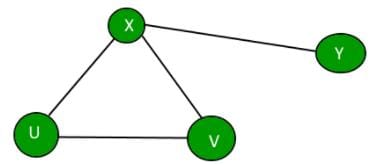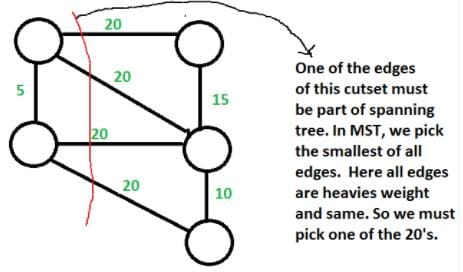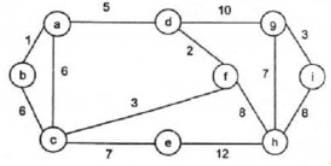# All India Computer Science Engineering (CSE) Group

## Which operator has the lowest priority?a)++b)%c)+d)||Correct answer is option 'D'. Can you explain this answer?

 Komal Pandey answered  •  1 week ago
D

## A binary search tree is generated by inserting in order the following integers: 50, 15, 62, 5, 20, 58, 91,3, 8, 37,60, 24. The number of nodes in the left subtree and right subtree of the root respectively isa)(4,7)b)(7,4)c)(8, 3)d)(3,8)Correct answer is option 'B'. Can you explain this answer?

 Vaibhavi Dyavarkonda answered  •  2 weeks ago
B

## Solve x=0.21 sin (0.5 x) by iteration method?

 Noor Noor answered  •  2 weeks ago
0.1224

## Find the Output ?#include "stdio.h"int main() {   int x, y = 5, z = 5;   x = y == z;   printf("%d", x);   getchar();   return 0; }a)0b)1c)5d)Compiler ErrorCorrect answer is option 'B'. Can you explain this answer?

 Naveen Kumar answered  •  3 weeks ago
In that statement they have considered equal operator ==
which means the value for x returns true if y and z are equal that indicates 1 otherwise 0

## Which of the following is not a type of database?a)Hierarchicalb)Networkc)Distributedd)DecentralizedCorrect answer is option 'D'. Can you explain this answer?

 Gate Gurus answered  •  3 weeks ago
Different types are:
1) Centralized
2) Distributed
3) Relational
4) NoSQL
5) Cloud
6) Object-oriented
7) Hierarchical
8) Network

## Assuming all numbers are in 2’s complement representation, which of the following numbers is divisible by 11111011?a)11100111b)11100100c)11010111d)11011011Correct answer is option 'A'. Can you explain this answer?

 Asif Khan answered  •  3 weeks ago
11100111

## In a trunk there are 3 types of ice cream of flavors vanilla, chocolate and blueberry. The probability of selecting one vanilla ice-cream is 12 and probability of selecting one blueberry ice-cream is 27. The total number of chocolate ice-cream is 6. Find the number of ice creams in the trunk:a)25b)30c)28d)32Correct answer is option 'C'. Can you explain this answer?

 Anbu Maran answered  •  4 weeks ago
28

## The descriptive property possessed by each entity set is _________a)Entityb)Attributec)Relationd)ModelCorrect answer is option 'B'. Can you explain this answer?

 Gate Gurus answered  •  4 weeks ago
Possible attributes of the instructor entity set are ID, name, dept name, and salary.

## An ________ is a set of entities of the same type that share the same properties, or attributes.a)Entity setb)Attribute setc)Relation setd)Entity modelCorrect answer is option 'A'. Can you explain this answer?

 Gate Gurus answered  •  4 weeks ago
An entity is a “thing” or “object” in the real world that is distinguishable from all other objects.

## The decoded instruction is stored in ______a)IRb)PCc)Registersd)MDRCorrect answer is option 'A'. Can you explain this answer?

 Rahul Bauri answered  •  4 weeks ago
What is sisd?

## What is the largest integer m such that every simple connected graph with n vertices and n edges contains at least m different spanning trees?a)1b)2c)3d)nCorrect answer is option 'C'. Can you explain this answer?

 Vibhor Goyal answered  •  4 weeks ago
A graph is connected iff all nodes can be traversed from each node. For a graph with n nodes, there will be n-1 minimum number of edges.
Given that there are n edges, that means a cycle is there in the graph.
The simplex graph with these conditions may be:Now we can make a different spanning tree by removing one edge from the cycle, one at a time.
Minimum cycle length can be 3, So, there must be atleast 3 spanning trees in any such Graph.

## Consider an undirected random graph of eight vertices. The probability that there is an edge between a pair of vertices is 1/2. What is the expected number of unordered cycles of length three?a)1/8b)1c)7d)8Correct answer is option 'C'. Can you explain this answer?

 Gate Gurus answered  •  4 weeks ago
A cycle of length 3 can be formed with 3 vertices. There can be total 8C3 ways to pick 3 vertices from 8. The probability that there is an edge between two vertices is 1/2. So expected number of unordered cycles of length 3 = (8C3)*(1/2)3 = 7

## Let G be a weighted undirected graph and e be an edge with maximum weight in G. Suppose there is a minimum weight spanning tree in G containing the edge e. Which of the following statements is always TRUE?a)There exists a cutset in G having all edges of maximum weight.b)There exists a cycle in G having all edges of maximum weightc)Edge e cannot be contained in a cycle.d)All edges in G have the same weightCorrect answer is option 'A'. Can you explain this answer?

 Gate Gurus answered  •  4 weeks ago
Background : Given a connected and undirected graph, a spanning tree of that graph is a subgraph that is a tree and connects all the vertices together.
1. A spanning tree has exactly V – 1 edges.
2. A single graph can have many different spanning trees. A minimum spanning tree (MST) or minimum weight spanning tree for a weighted, connected and undirected graph is a spanning tree with weight less than or equal to the weight of every other spanning tree. The weight of a spanning tree is the sum of weights given to each edge of the spanning tree.
3. There can be more that one possible spanning trees of a graph. For example, the graph in this question has 6 possible spanning trees.
4. MST has lightest edge of every cutset. Remember Prim’s algorithm which basically picks the lightest edge from every cutset.
Choices of this question :
a) There exists a cutset in G having all edges of maximum weight : This is correct. If there is a heaviest edge in MST, then there exist a cut with all edges with weight equal to heavies edge. See point 4 discussed in above background.b) There exists a cycle in G having all edges of maximum weight : Not always TRUE. The cutset of heaviest edge may contain only one edge. In fact there may be overall one edge of heavies weight which is part of MST (Consider a graph with two components which are connected by only one edge and this edge is the heavies)
c) Edge e cannot be contained in a cycle. Not Always True. The curset may form cycle with other edges.
d) All edges in G have the same weight Not always True. As discussed in option b, there can be only one edge of heaviest weight.

## Which kind of traversal order trie gives the lexicographical sorting of the set of the strings?a)Preorderb)Postorderc)Level orderd)InorderCorrect answer is option 'A'. Can you explain this answer?

 Gate Gurus answered  •  4 weeks ago
To print the string in alphabetical order we have to first insert in the trie and then perform preorder traversal to print in alphabetical order.

## The cyclomatic complexity of the flow graph of a program provides a)an upper bound for the number of tests that must be conducted to ensure that all statements have been executed at most onceb)a lower bound for the number of tests that must be conducted to ensure that all statements have been executed at most oncec)an upper bound for the number of tests that must be conducted to ensure that all statements have been executed at least onced)a lower bound for the number of tests that must be conducted to ensure that all statements have been executed at least onceCorrect answer is option 'C'. Can you explain this answer?

 Gate Gurus answered  •  4 weeks ago
Option (C) is correct, because the cyclomatic complexity of the flow graph of a program provides an upper bound for the number of tests that must be conducted to ensure that all statements have been executed at least once.

## Let G be a simple graph with 20 vertices and 8 components. If we delete a vertex in G, then number of components in G should lie between ____.a)8 and 20b)8 and 19c)7 and 19d)7 and 20Correct answer is option 'C'. Can you explain this answer?

 Gate Gurus answered  •  4 weeks ago
Case 1: If the vertex we are deleting from G is an isolated vertex, which is a component by itself, then number of components in G becomes 7.
Case 2: If G is a start Graph, then by deleting the cut vertex of G, we get 19 components.
Hence, number of components in G should lie between 7 and 19.

## What is the maximum number of edges in an acyclic undirected graph with n vertices?a)n-1b)nc)n + 1d)2n-1Correct answer is option 'A'. Can you explain this answer?

 Gate Gurus answered  •  4 weeks ago
n * (n – 1) / 2 when cyclic. But acyclic graph with the maximum number of edges is actually a spanning tree and therefore, correct answer is n-1 edges.

## Given an undirected graph G with V vertices and E edges, the sum of the degrees of all vertices isa)Eb)2Ec)Vd)2VCorrect answer is option 'B'. Can you explain this answer?

 Machine Experts answered  •  4 weeks ago
Since the given graph is undirected, every edge contributes as 2 to sum of degrees.
So the sum of degrees is 2E.

## Which of the following data structure is useful in traversing a given graph by breadth first search?a)Stackb)Listc)Queued)None of the above.Correct answer is option 'C'. Can you explain this answer?

 Machine Experts answered  •  4 weeks ago
BFS performs level-order traversal which can be fairly done using a queue. A queue uses FIFO ordering and the nodes that we enqueue first are explored first maintaining the order of traversal.

## You are given a graph containing n vertices and m edges and given that the graph doesn’t contain cycle of odd length. Time Complexity of the best known algorithm to find out whether the graph is bipartite or not is ?a)O(m+n)b)O(1)c)O(mn)d)O(n2)Correct answer is option 'B'. Can you explain this answer?

 Machine Experts answered  •  4 weeks ago
It is by definition that a graph is bipartite iff it does not contain odd length cycles.

## For the undirected, weighted graph given below, which of the following sequences of edges represents a correct execution of Prim’s algorithm to construct a Minimum Span­ning Tree?a)(a, b), (d, f), (f, c), (g, i), (d, a), (g, h), (c, e), (f, h)b)(c, e), (c, f), (f, d), (d, a), (a, b), (g, h), (h, f), (g, i)c)(d, f), (f, c), (d, a), (a, b), (c, e), (f, h), (g, h), (g, i)d)(h, g), (g, i), (h, f), (f, c), (f, d), (d, a), (a, b), (c, e)Correct answer is option 'C'. Can you explain this answer?

 Machine Experts answered  •  4 weeks ago
In prims algorithm we start with any node and keep on exploring minimum cost neighbors of nodes already covered.